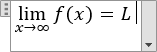# How to insert an equation with a limit

Word
This tip shows how to add an equation with a limit. For example, the formula of a limit.

How to add an equation in your document, see Working with Microsoft Equation.

## In the Professional format:

2.   On the Equation tab, in the Structures group, click the Limit and Log drop-down list: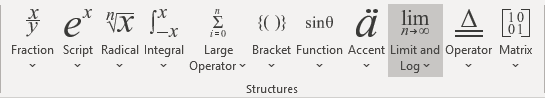In the Limit and Log list, choose Limit: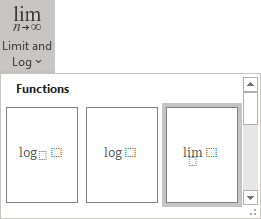3.   In the limit template:

• In the lower box, enter:
• x,
• On the Equation tab, in the Symbols group, in the list of symbols, choose Right Arrow: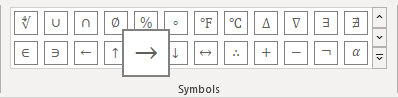• On the Equation tab, in the Symbols group, in the list of symbols, choose Infinity: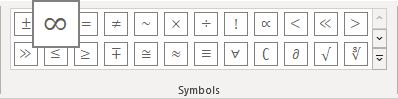• In the right base box, enter f(x).

4.   Then enter = L: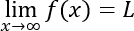## In the Linear format:

2.   Enter one of the following:

• lim_(x ->
• lim_(x \to
• lim_(x \rightarrow

Then you enter a space key, this linear formula transformed to the professional format: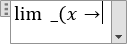3.   Enter \infty: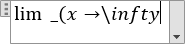After clicking a space key, Word will show an infinity symbol: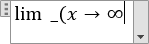4.   Enter ) and a space key: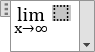5.   In the base box of the limit, enter f (x).

6.   Enter = L: